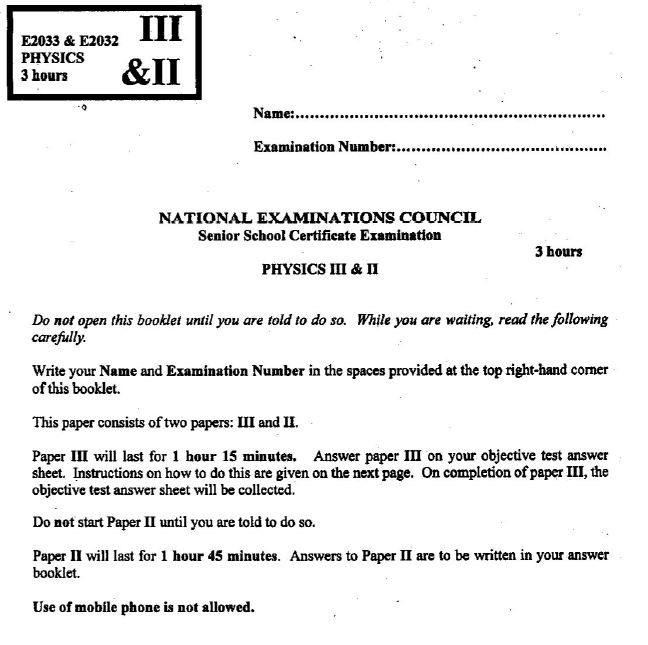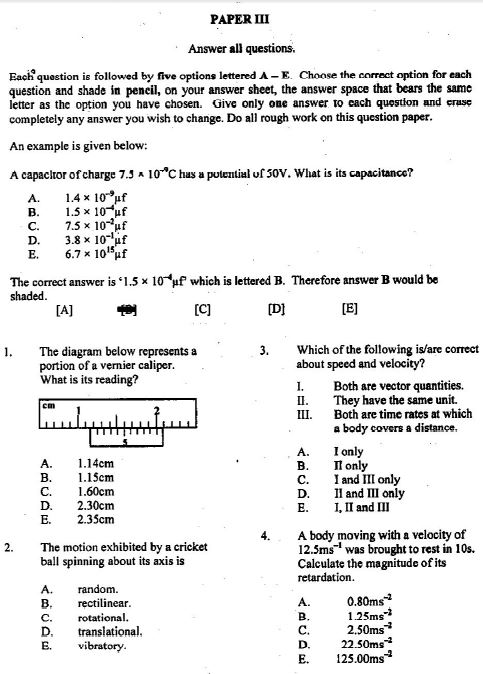# NECO Physics Answers 2019 for Obj-Essay Questions is Out

## 2019 NECO Physics Answers for SSCE Questions Released.

The Neco Physics answers 2019 is now available. The National Examination Council (NECO) Senior School Certificate Examination (SSCE) Physics paper will be written on Thursday 20th June, 2019.

The o’level Neco physics questions below is for Paper III & II: Objective & Essay which will commence from 10.00am and end by 01.00pm. That means the examination will last for three hours.

The resources below on Neco Physics questions 2019 have been provided to assist you understand the required standards expected in NECO Physics final Examination. Continue reading.### NECO Physics Answers 2019:

1. A stone projected horizontally from the top of a tower with a speed of 4 ms-1 lands on the level ground at a horizontal distance of 25 m from the foot of the tower. Calculate the height  of the tower. [ g = 10 ms-2 ]

2. (a) Explain diffusion.
(b) Give one reason why the rate of diffusion is higher in gasses than in liquid at the same temperature.

3. (a) State two conditions under which photo-electrons can be emitted from the surface of a metal.
(b) List two particles characteristics of electromagnetic waves.
ANS: (a) Conditions for emission of photoelectrons
– the wavelength of incident radiation must be less than the threshold wavelength of metal surface.
– the frequency of incident radiation must be greater than the threshold frequency.
– the energy of the incident photons (radiation) must be greater than the Work function of the metal
(b) Particle characteristics of electromagnetic waves;
e.g – Photoelectric effect
– Thermionic emission
– Radiations from heated bodies (Black body)
– Emission and absorption of light  (incandescence)
– Compton effect.

4. (a) Explain how a gas can be made to conduct electricity.
(b) Name the electric charge carriers in gases.
ANS: (a) The molecules /atoms of a gas must be ionized  before the gas can conduct electricity. Ionisation of the gas requires that the gas pressure is very low within the enclosure and a very high voltage is applied to the enclosed gas.
(b) Charge carries in gases are Electrons; Ions

5. State;
(a) two applications of electrolysis in an industry;
(b) one application of electrolysis in a school laboratory.

6. Explain why water in a narrow glass tube has a concave meniscus while mercury, in the same tube, has a convex meniscus.

7. (a) (i) What is an eclipse?
(ii) List the three types of eclipse.
(b) A student in a lecture theatre can read from the board clearly but requires a pair of spectacles to read from a book.
(i) What eye defect has this student?
(ii) What type of lens is needed to correct the eye defect?
(iii) The focal length of the lens used to correct this defect is 10 cm. Calculate the   power of the lens.
(c) A car B moves towards a stationary car A.  If B produces an ultrasonic sound at a point and it takes 5.6 x 10-3  s for a beep to be heard in B, calculate the distance between the two cars at that instant. (Speed of sound in air = 340 ms-1)
(d) The image of an object is located 9 cm behind a convex mirror.  If the magnification produced is 0.6, calculate the focal length of the mirror.

8. (a) (i) What is a vector quantity?
(ii) Three vectors 3 ms-1 N 45o W, 12 ms-1W and 5ms-1 S act at a point.
(iii) Sketch a vector diagram to illustrate the given information.
(iv) Calculate the resultant of the vectors.
(b) In a laboratory experiment to determine the force constant of a spiral spring, the mass on the spring was varied and the corresponding extensions were measured and recorded as shown in the table below.

 Mass m/g Weight W/N Extension e/cm 50 100 150 200 250 6.5 11.0 15.0 20.0 25.0

(i) Copy and complete the table.  (Take g = 10ms-2 )
(ii) Plot a graph with weight, W, on the vertical axis and extension, e, on the horizontal axis.
(iii) Using the graph, determine the force constant of the spring.
(iv) Determine the natural length of the spring if its length was 38.0 cm when loaded with 250 g mass.

9. (a) Define heat capacity and state its unit.
(b) List two effects of heat on a substance
(c) Explain how a tightly fitted glass stopper could be removed from a reagent bottle.
(d) A quantity of pepper soup of mass 800g poured into a plastic container with a tight fitting lid has a temperature of 30oC. The container is then placed in a microwave oven, rated 1200 W and operated for 3 minutes.
(i) Calculate the final temperature attained by the soup.  (Assuming no heat losses).
(ii) Explain why containers with tight-fitting lids are not suitable for use in microwave cooking.
(iii) When the soup is brought out and allowed to cool, a dent was observed on the container. Explain.
[ Take specific heat capacity of the soup  =  4000 Jkg-1 K-1 ]

10. (a) State the three characteristics of sound and the factor on which each of them depends.
(b) Explain resonance as applied to sound.
(c) What role does echo play in the construction of a concert hall?
(d) The surface of an ear drum (assumed circular) has a radius 2.1 mm.  It resonates with an amplitude of 0.8 x 10-7 m as a result of impulses received from an external body vibrating at 2400 Hz. If the resulting pressure change on the ear drum is 3.6 x 10-5 Nm-2. Calculate the:
(i) period of oscillation
(ii) velocity
(iii) acceleration
(iv) force.
[Pi =  3.14]

11. (a) Define electromotive force.
(b) State:
(i) the principle of operation of a potentiometer;
(ii) two advantages that a potentiometer has over a voltmeter in measuring potential difference.
(c) (i) Sketch and label a diagram of a gold-leaf electroscope.
(ii) Give one use of a gold-leaf electroscope.
(d) (i) Explain the action of a magnetic relay:
(ii) List two factors which determine the magnitude of an induced emf in a coil.
(iii) A current of 5 A passes through a straight wire in a uniform magnetic field of flux density 2.0 x 10-3 T.  Calculate the force per unit length exerted on the wire when it is inclined at 30o to the field.

12.

#### Neco Physics Objective Questions:5.

Note: There is nothing like Neco Physics Expo online. Neco Ssce candidates are to desist from patronizing online fraudsters / vendors who says they can provide such services as they are not real.

Spread the love - Share this with your friends

### Comments (0)

This site uses Akismet to reduce spam. Learn how your comment data is processed.